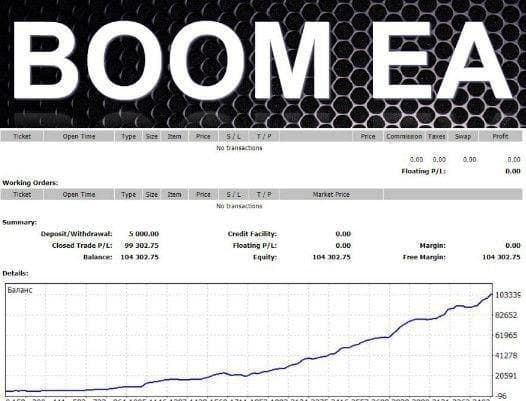# BOOM EA Expert

Date: Nov 24th 20BOOM EA Expert

The Expert Advisor is based on the indicator analysis. Trading decisions are made using Moving Average values. Indicators can be configured by external variables:

• TF = 0 - select a working timeframe. It can be one of the chart periods. 0 means the current chart period. 1 - 1 minute. 5 - 5 minutes. 15 - 15 minutes. 30 - 30 minutes. 60 - 1 hour. 240 - 4 hours. 1440 - 1 day. 10 080 - 1 week. 43 200 - 1 month.
• shift = 0 - index of obtained value from the indicator buffer (shifting back by specified periods relative to the current bar).
• period_fast = 5 - Fast Moving Average period.
• period_slow = 12 - Slow Moving Average period.
• mehod = 0 - averaging method. It can have any values ​​of the Moving Average method (Moving Average). 0 - (SMA) Simple Moving Average. 1 - (EMA) Exponential Moving Average. SMMA 2 - (SMMA) Smoothed Moving Average. LWMA 3 - (LWMA) Linear weighed Moving Average.
• applied = 0 - applied price. It can be any of the price constants. 0 - (CLOSE) Close price. 1 - (OPEN) Open price. 2 - (HIGH) maximum price. 3 - (LOW) minimum price. 4 - (MEDIAN) average price, (high+low)/2. 5 - (TYPICAL) typical price, (high+low+close)/3. 6 - (WEIGHTED) weighted close price, (high+low+close+close)/4.
• MagicNumber = 2808 - order magic number.

Restrictive operation by time is used as a filter in the EA operation. The settings are as follows:

• StartHour=07 - operation start hours
• StartMinute=01 - operation start minutes
• StopHour=19 - operation stop hours
• StopMinute=59 - operation stop minutes

Each opened position is protected against drawdown by a "lock". It can be configured as follows:

• step_lock = 25 - distance in points before opening the next locking order
• koef_lot = 2 - lot ratio for locking positions
• Profit_Plus = 30 - profit level when exiting a drawdown

The EA also allows you to configure loss and profit levels for main positions, money management and trailing open positions.

• StopLoss = 0 - loss level in points
• TakeProfit = 0 - profit level in points
• Lots = 0.1 - forced deal volume; if 0, ММ is enabled
• Choice_method = false - select ММ method based on free funds or the balance
• Risk = 0.3 - ММ risk volume, lot auto calculation
• MarketWatch = False - set profit and loss levels by modification
• Slippage = 30 - slippage level
• TSProfitOnly = true - switch the zone where trailing is to start working. If True, trailing starts working only when position profit reaches amount of points equal to TStop_Buy/Sell+TrailingStep. If False, the EA simply ensures that a stop level relative to the current price is always not further than TStop_Buy/Sell+TrailingStep amount of points. In other words, if True, the EA works only in a profitable position area, while if False, in a negative, as well.